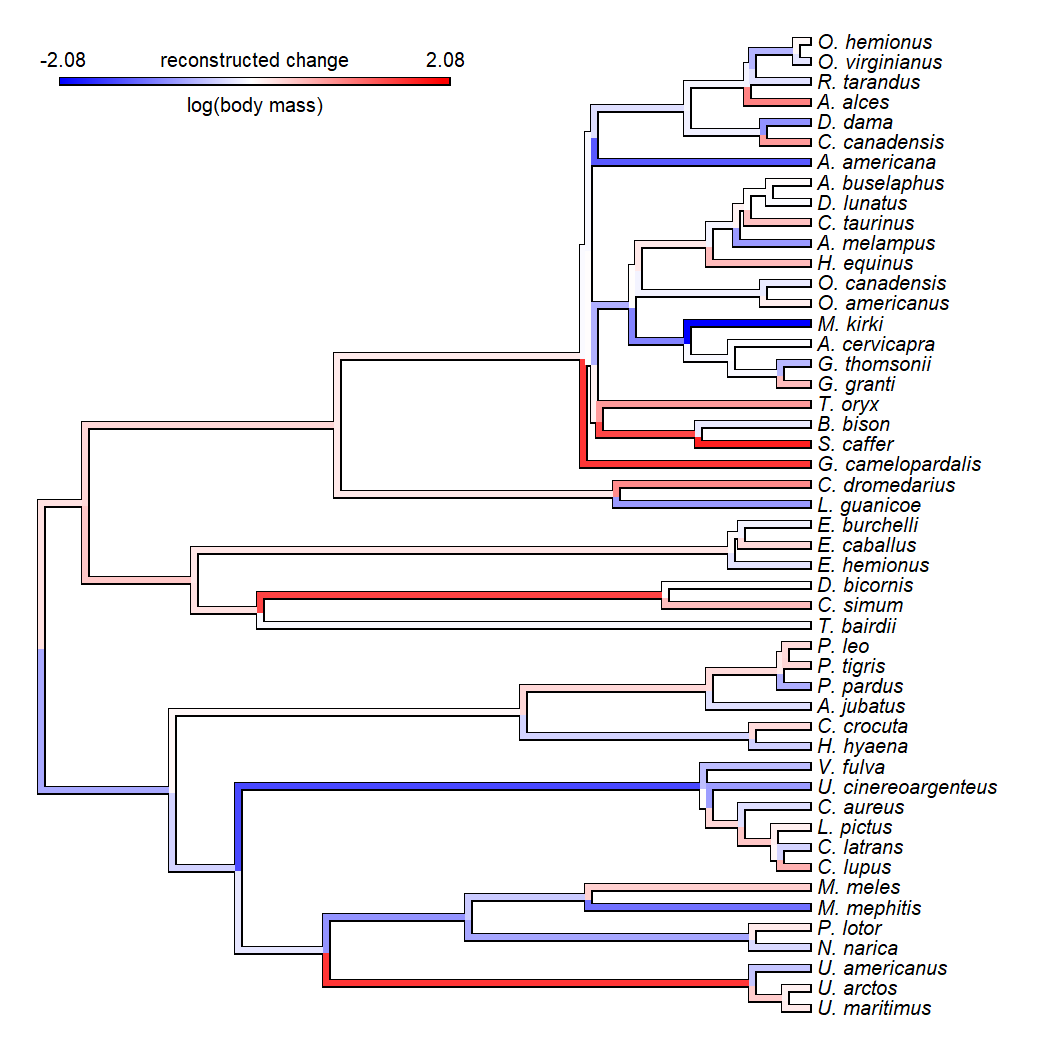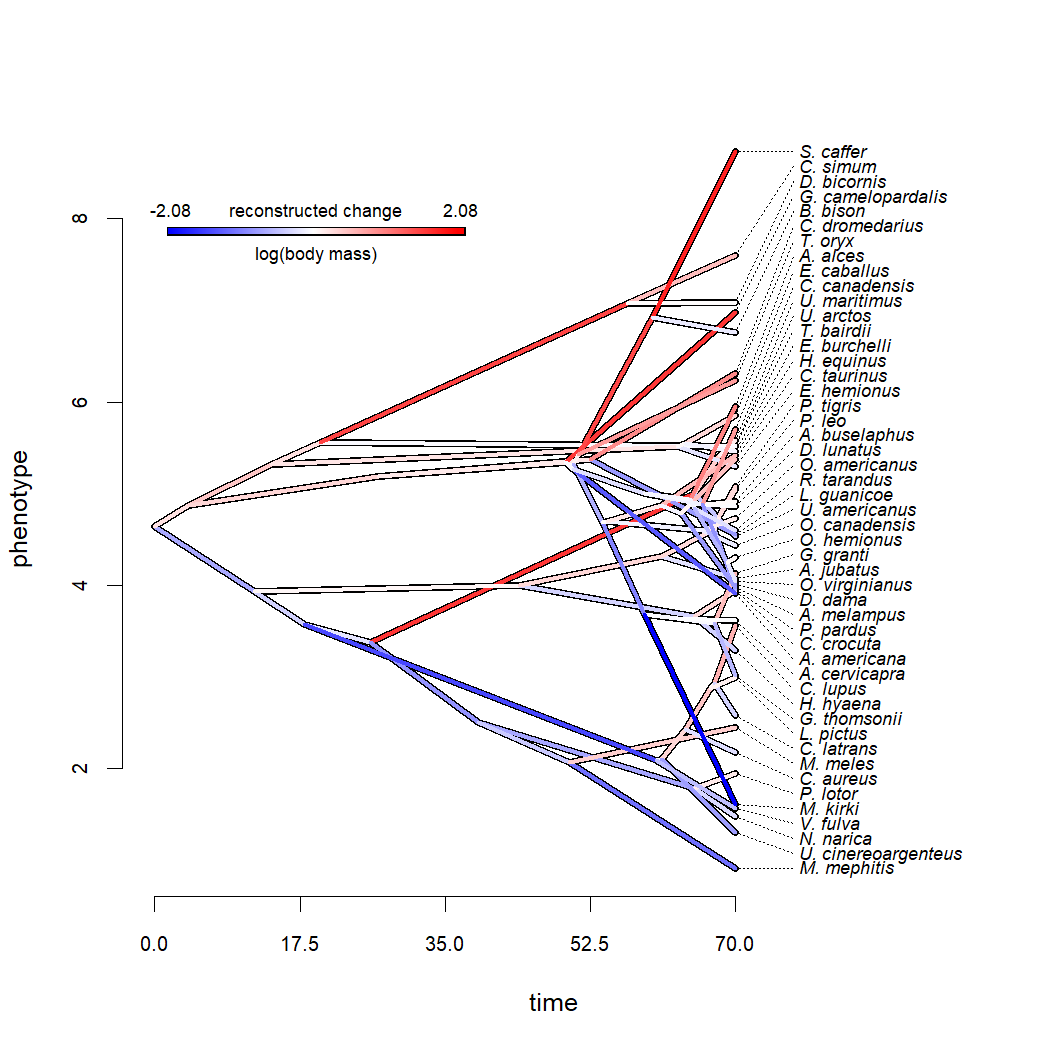## Monday, May 3, 2021

### Computing (& plotting) the implied changes from ancestral state reconstruction on the branches of a phylogeny

“I am using phytools to reconstruct ancestral states of a continuous trait on a phylogenetic tree, and was wondering if there is a function in phytools to calculate the number of branches that show an increase in trait value and the number that show a decrease? And if so, if there's a way to visualise this, such as all branches showing an increase in one colour and all showing a decrease in another colour?”

Without advising specifically on whether or not ancestral state reconstructions should be used in this way (I choose to remain agnostic on this point), the answer is, of course 'yes' – this can absolutely be done in R. (To paraphrase Simone Blomberg, with R it's a question of how not if.)

To illustrate this, I will use data for body mass in mammals that come from Garland et al. (1992). Let's load these data, and then estimate ancestral states using `fastAnc`.

``````library(phytools)
data(mammal.tree)
data(mammal.data)
lnMass<-setNames(log(mammal.data\$bodyMass),
rownames(mammal.data))
fit<-fastAnc(mammal.tree,lnMass)
fit
``````
``````## Ancestral character estimates using fastAnc:
##       50       51       52       53       54       55       56       57
## 4.640573 3.941365 3.580030 3.378235 5.008602 5.417042 2.506860 1.783328
##       58       59       60       61       62       63       64       65
## 2.075654 2.092616 2.102696 2.427963 2.879837 2.935833 4.003930 3.660685
##       66       67       68       69       70       71       72       73
## 4.315032 4.607959 4.760302 4.873642 5.317474 5.559303 7.078588 5.517309
##       74       75       76       77       78       79       80       81
## 5.552671 5.192977 5.370860 5.348416 5.312073 5.325223 5.429285 6.920095
##       82       83       84       85       86       87       88       89
## 4.688839 3.685079 3.636850 3.590634 4.698303 4.603683 4.874816 4.790504
##       90       91       92       93       94       95       96       97
## 4.882241 4.886430 5.262581 5.009917 4.889860 4.940759 4.842665 4.247903
``````

Alright. Now, first, how do we simply count the number of edges that involve a recontructed increase in log body mass, compared to those involved an estimated decrease?

This is probably a lot easier than you'd think.

We just need to remember the structure of our `"phylo"` object.

In a `"phylo"` object, the branches of the tree are encoded in a matrix (called `edge`) in which each row contains the starting and ending edge indices of each branch in the phylogeny. If we paste together our original data values (in the order of the tip labels of the phylogeny) and our estimated ancestral states (in the order of increasing node index value), then we'll have a vector containing the observed or reconstructed trait value for each tip and node of the phylogeny, in the order of the node & tip indices. We can then simply restructure this vector into a matrix based on the indices in `edge`.

``````node.vals<-mammal.tree\$edge
node.vals[]<-c(lnMass[mammal.tree\$tip.label],
fit)[mammal.tree\$edge]
``````

Let's look at the first 10 rows of this matrix:

``````head(node.vals,10)
``````
``````##           [,1]     [,2]
##  [1,] 4.640573 3.941365
##  [2,] 3.941365 3.580030
##  [3,] 3.580030 3.378235
##  [4,] 3.378235 5.008602
##  [5,] 5.008602 5.417042
##  [6,] 5.417042 5.579730
##  [7,] 5.417042 5.526647
##  [8,] 5.008602 4.536891
##  [9,] 3.378235 2.506860
## [10,] 2.506860 1.783328
``````

Cool, now let's proceed to simply count the edges over which the trait increased:

``````increases<-sum(node.vals[,2]>node.vals[,1])
increases
``````
``````##  50
``````

This tells is that on 50 edges of the tree, the trait log(body mass) is increasing, according to our reconstruction.

Every (non zero-length) edge must involve an increase or a decrease, so the decreases should be just the number of edges in our tree (96) minus this value (i.e., 46), but let's check just to be sure:

``````decreases<-sum(node.vals[,2]<node.vals[,1])
decreases
``````
``````##  46
``````

The next part of the question was if there is “a way to visualise this, such as all branches showing an increase in one colour and all showing a decrease in another colour”.

Of course we can do this – but I'd say that we can actually do even better.

What I'm going to do is create a custom color gradient such that red is a large positive change; blue is a large negative change; and white is no change at all. Then I'm going to plot the tree with these colors.

Let's see what that looks like:

``````cols<-setNames(
colorRampPalette(c("blue","white","red"))(510),
1:510)
edge.vals<-node.vals[,2]-node.vals[,1]
max.val<-max(abs(edge.vals))+1e-8
intervals<-seq(-max.val,max.val,length.out=511)
intervals<-cbind(intervals[1:510],intervals[2:511])
edge.ind<-sapply(edge.vals, function(x,intervals)
intersect(which(x>intervals[,1]),which(x<=intervals[,2])),
intervals=intervals)
painted<-paintBranches(mammal.tree,mammal.tree\$edge[1,2],
state=edge.ind)
for(i in 2:length(edge.ind))
painted<-paintBranches(painted,mammal.tree\$edge[i,2],
state=edge.ind[i])
plot(painted,colors=cols,lwd=4,split.vertical=TRUE,
outline=TRUE,ftype="i",fsize=0.8)
title="reconstructed change",subtitle="log(body mass)",
lims=c(-max.val,max.val),digits=2,prompt=FALSE,x=2,
y=Ntip(mammal.tree)-2,fsize=0.8)
``````Pretty cool.

For fun, let's take a look at how this appears on a traitgram. Edges going up (that is, towards increasing values for the trait) should be in red, edges going down should be in blue.

Let's check.

``````par(cex.axis=0.8)
lwd=5)
``````
``````## Optimizing the positions of the tip labels...
``````
``````par(fg="transparent")
``````
``````## Optimizing the positions of the tip labels...
``````
``````par(fg="black")
title="reconstructed change",subtitle="log(body mass)",
lims=c(-max.val,max.val),digits=2,prompt=FALSE,x=2,
y=0.9*max(lnMass),fsize=0.7)
``````OK, that's pretty neat, right?

#### 1 comment:

1.Hi Liam,
Quite impressive!
would it be possible to do so with morphometric results of a PCA? Is so how to do it?
Eric

Note: due to the very large amount of spam, all comments are now automatically submitted for moderation.